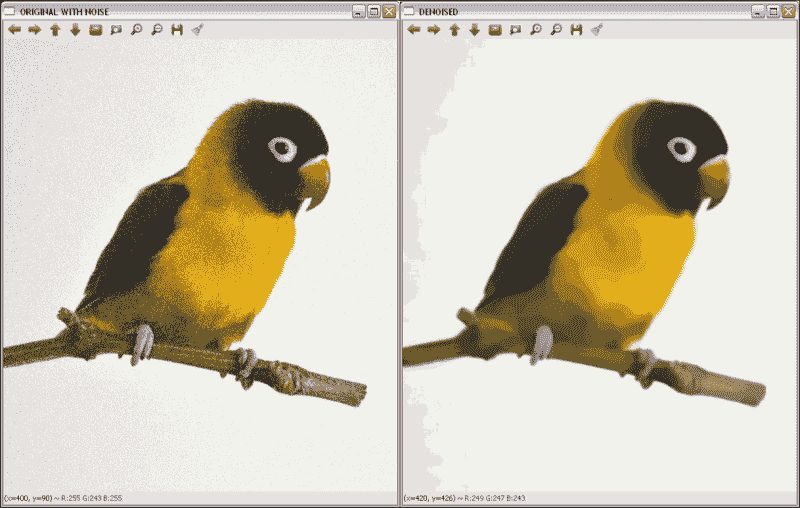# 三、校正和增强图像

• 图像过滤。 这包括图像平滑，图像锐化以及使用图像金字塔。
• 应用形态学操作，例如扩张，腐蚀，打开或关闭。
• 几何变换（仿射和透视变换）。
• 修复，用于重建图像的受损部分。
• 去噪，这对于减少图像捕获设备产生的图像噪声是必需的。

# 图像过滤

OpenCV 为常见的图像处理操作（例如平滑或锐化）提供几种过滤功能。

## 平滑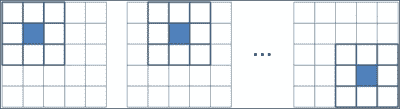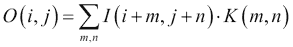• void boxFilter(InputArray src, OutputArray dst, int ddepth, Size ksize, Point anchor = Point(-1,-1), bool normalize = true, int borderType = BORDER_DEFAULT)：这是一个盒子过滤器，其核系数相等。 使用normalize=true时，每个输出像素值都是其核邻居的平均值，所有系数均等于1 / n，其中n为元素数。 使用normalize=false时，所有系数都等于 1。src参数是输入图像，而滤波后的图像存储在dst中。 ddepth参数指示输出图像深度为 -1，以使用与输入图像相同的深度。 核大小在ksize中指示。 anchor点指示所谓的锚点像素的位置。 默认值(-1, -1)表示锚点位于核的中心。 最后，在borderType参数中指示边界类型的处理。
• void GaussianBlur(InputArray src, OutputArray dst, Size ksize, double sigmaX, double sigmaY = 0, int borderType=BORDER_DEFAULT): This is done by convolving each point in the src input array with a Gaussian kernel to produce the dst output. The sigmaX and sigmaY parameters indicate the Gaussian kernel standard deviation in X and Y directions. If sigmaY is zero, it is set to be equal to sigmaX, and if both are equal to zero, they are computed using the width and height given in ksize.

### 注意

卷积定义为两个函数乘积的积分，其中两个函数之一先前已被反转和移位。

• void medianBlur(InputArray src, OutputArray dst, int ksize)：这遍历图像的每个元素，并将每个像素替换为其相邻像素的中间值。

• void bilateralFilter(InputArray src, OutputArray dst, int d, double sigmaColor, double sigmaSpace, int borderType=BORDER_DEFAULT)：这类似于高斯过滤器，其中考虑了相邻像素，每个像素都分配有权重，但每个权重上都有两个分量，这与高斯过滤器使用的分量相同，而另一个考虑了相邻像素和评估像素之间的强度。 此函数需要像素邻域的直径作为参数dsigmaColor sigmaSpace值。 sigmaSpace参数的较大值表示像素邻域内的其他颜色将混合在一起，从而生成较大的半均等颜色区域，而sigmaSpace参数的较大值表示较远像素将彼此影响。 只要它们的颜色足够接近。
• void blur(InputArray src, OutputArray dst, Size ksize, Point anchor=Point(-1,-1), int borderType=BORDER_DEFAULT): This blurs an image using the normalized box filter. It is equivalent to using boxFilter with normalize = true. The kernel used in this function is: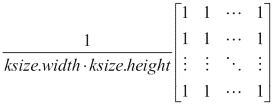### 注意

getGaussianKernelgetGaborKernel函数可以在 OpenCV 中使用以生成自定义核，然后可以将其传递给filter2D

• BORDER_REPLICATE：此操作重复上一个已知的像素值：aaaaaa | abcdefgh | hhhhhhh
• BORDER_REFLECT：这反映了图像边框：fedcba | abcdefgh | hgfedcb
• BORDER_REFLECT_101：这将反映图像边框，而不复制边框的最后一个像素：gfedcb | abcdefgh | gfedcba
• BORDER_WRAP：这将追加相对边框的值：cdefgh | abcdefgh | abcdefg
• BORDER_CONSTANT：这将在新边界上建立一个常数：kkkkkk | abcdefgh | kkkkkk

### 示例代码

#include "opencv2/opencv.hpp"

using namespace cv;

int main( int argc, char** argv )
{
Mat src;

// Apply the filters
Mat dst, dst2;
GaussianBlur( src, dst, Size( 9, 9 ), 0, 0);
medianBlur( src, dst2, 9);

// Show the results
namedWindow( " ORIGINAL ", WINDOW_AUTOSIZE );
imshow( " ORIGINAL ", src );
namedWindow( " GAUSSIAN BLUR ", WINDOW_AUTOSIZE );
imshow( " GAUSSIAN BLUR ", dst );
namedWindow( " MEDIAN BLUR ", WINDOW_AUTOSIZE );
imshow( " MEDIAN BLUR ", dst2 );

waitKey();
return 0;
}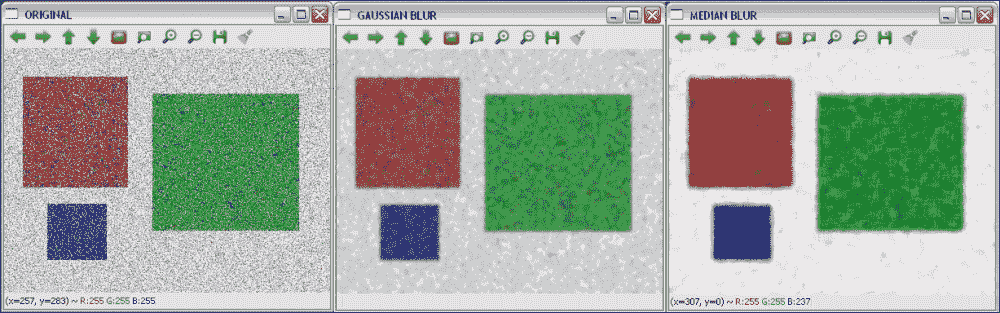## 锐化

Sobel 运算符通过以下方式计算图像I的一阶图像导数：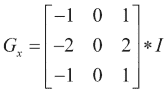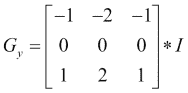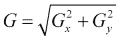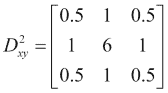• void Sobel(InputArray src, OutputArray dst, int ddepth, int dx, int dy, int ksize = 3, double scale = 1, double delta = 0, int borderType = BORDER_DEFAULT)：这将根据src中的图像使用 Sobel 运算符计算一阶，二阶，三阶或混合图像导数。 ddepth参数指示输出图像深度，即 -1 至使用与输入图像相同的深度。 籽粒大小在ksize中指示，所需的导数阶数在dxdy中指示。 可以使用scale建立计算得出的微分值的比例因子。 最后，在borderType参数中指示边界类型处理，并且可以在将结果存储在dst中之前将delta值添加到结果中。
• void Scharr(InputArray src, OutputArray dst, int ddepth, int dx, int dy, double scale = 1, double delta = 0, int borderType = BORDER_DEFAULT )：这为大小为3 x 3的核计算了一个更准确的导数。Scharr(src, dst, ddepth, dx, dy, scale, delta, borderType)等效于Sobel(src, dst, ddepth, dx, dy, CV_SCHARR, scale, delta, borderType)
• void Laplacian(InputArray src, OutputArray dst, int ddepth, int ksize = 1, double scale = 1, double delta = 0, int borderType = BORDER_DEFAULT)：这将计算图像的拉普拉斯算子。 除了ksize以外，所有参数均与SobelScharr函数中的参数相同。 当ksize >为 1 时，它通过将使用Sobel计算的第二xy导数相加来计算src中图像的拉普拉斯算子。 当ksize = 1时，通过用3 x 3核对图像进行滤波来计算拉普拉斯算子，该核包含 -4 为中心，0 为角，其余为 1。

### 注意

getDerivKernels可以在 OpenCV 中使用以生成自定义的派生核，然后可以将其传递给sepFilter2D

### 示例代码

#include "opencv2/opencv.hpp"

using namespace cv;

int main( int argc, char** argv )
{
Mat src;

// Apply Sobel and Laplacian
Mat dst, dst2;
Sobel(src, dst, -1, 1, 1 );
Laplacian(src, dst2, -1 );

// Show the results
namedWindow( " ORIGINAL ", WINDOW_AUTOSIZE );
imshow( " ORIGINAL ", src );
namedWindow( " SOBEL ", WINDOW_AUTOSIZE );
imshow( " SOBEL ", dst );
namedWindow( " LAPLACIAN ", WINDOW_AUTOSIZE );
imshow( " LAPLACIAN ", dst2 );

waitKey();
return 0;
}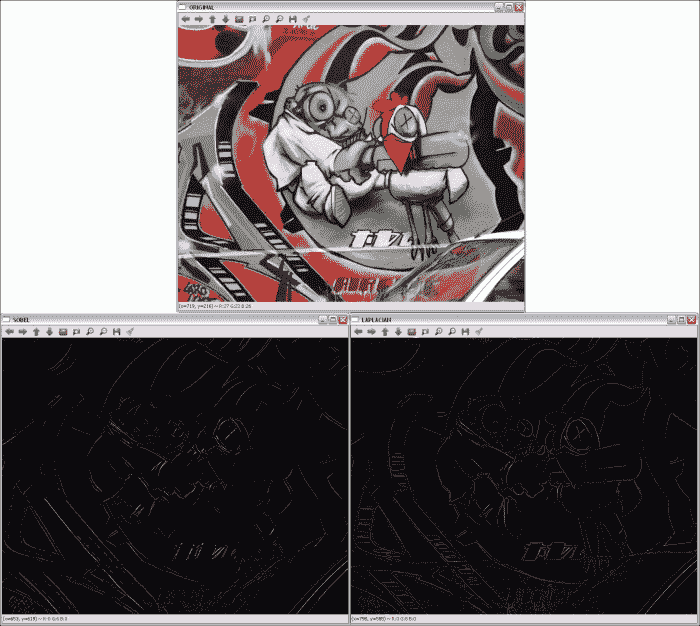## 使用图像金字塔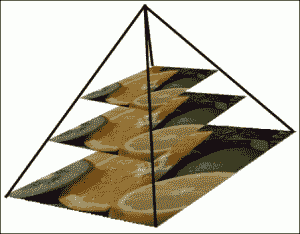### 高斯金字塔

• void pyrDown(InputArray src, OutputArray dst, const Size& dstsize = Size(), int borderType = BORDER_DEFAULT)：此子采样会模糊src图像，并将结果保存在dst中。 如果未使用dstsize参数设置输出图像的大小，则将其计算为Size((src.cols+1)/2, (src.rows+1)/2)
• void pyrUp(InputArray src, OutputArray dst, const Size& dstsize = Size(), int borderType = BORDER_DEFAULT)：计算pyrDown的相反过程。
• void buildPyramid(InputArray src, OutputArrayOfArrays dst, int maxlevel, int borderType = BORDER_DEFAULT)：这将为src中存储的图像构建高斯金字塔，获取maxlevel新图像，然后将它们存储在dst中原始图像之后的dst数组中。 因此，dst结果存储了maxlevel + 1图像。

• void pyrMeanShiftFiltering(InputArray src, OutputArray dst, double sp, double sr, int maxLevel = 1, TermCriteria termcrit = TermCriteria (TermCriteria::MAX_ITER + TermCriteria::EPS, 5, 1)): This implements the filtering stage of the mean-shift segmentation, obtaining an image, dst, with color gradients and fine-grain texture flattened. The sp and sr parameters indicate the spatial window and the color window radii.

### 注意

可以在这个页面中找到有关均值漂移分割的更多信息。

### 示例代码

#include "opencv2/opencv.hpp"

using namespace cv;

int main( int argc, char** argv )
{
Mat src;

// Apply two times pyrDown
Mat dst, dst2;
pyrDown(src, dst);
pyrDown(dst, dst2);

// Show the results
namedWindow( " ORIGINAL ", WINDOW_AUTOSIZE );
imshow( " ORIGINAL ", src );
namedWindow( " 1st PYRDOWN ", WINDOW_AUTOSIZE );
imshow( " 1st PYRDOWN ", dst );
namedWindow( " 2st PYRDOWN ", WINDOW_AUTOSIZE );
imshow( " 2st PYRDOWN ", dst2 );

// Apply two times pyrUp
pyrUp(dst2, dst);
pyrUp(dst, src);

// Show the results
namedWindow( " NEW ORIGINAL ", WINDOW_AUTOSIZE );
imshow( " NEW ORIGINAL ", dst2 );
namedWindow( " 1st PYRUP ", WINDOW_AUTOSIZE );
imshow( " 1st PYRUP ", dst );
namedWindow( " 2st PYRUP ", WINDOW_AUTOSIZE );
imshow( " 2st PYRUP ", src );

waitKey();
return 0;
}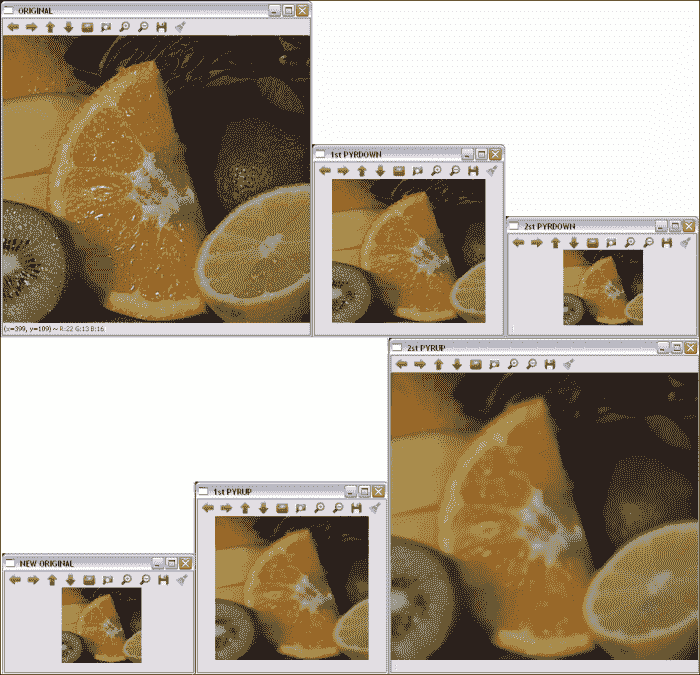# 形态操作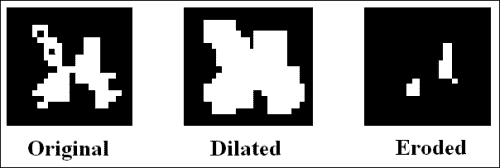• void dilate(InputArray src, OutputArray dst, InputArray kernel, Point anchor = Point(-1,-1), int iterations = 1, int borderType = BORDER_CONSTANT, const Scalar& borderValue = morphologyDefaultBorderValue())：这会使用特定的结构化元素扩大src中存储的图像，并将结果保存在dst中。 kernel参数是所使用的结构元素。 anchor点指示锚点像素的位置。 (-1, -1)值表示锚点位于中心。 使用iterations可以多次应用该操作。 边界类型的处理在borderType参数中指示，与前面部分中的其他过滤器相同。 最后，如果使用BORDER_CONSTANT边界类型，则在borderValue中指示常量。
• void erode(InputArray src, OutputArray dst, InputArray kernel, Point anchor = Point(-1,-1), int iterations = 1, int borderType = BORDER_CONSTANT, const Scalar& borderValue = morphologyDefaultBorderValue())：这会使用特定的结构元素腐蚀图像。 其参数与dilate中的参数相同。
• void morphologyEx(InputArray src, OutputArray dst, int op, InputArray kernel, Point anchor = Point(-1,-1), int iterations = 1, int borderType = BORDER_CONSTANT, const Scalar& borderValue = morphologyDefaultBorderValue())：这执行使用op参数定义的高级形态学操作。 可能的op值为MORPH_OPENMORPH_CLOSEMORPH_GRADIENTMORPH_TOPHATMORPH_BLACKHAT
• Mat getStructuringElement(int shape, Size ksize, Point anchor = Point(-1,-1))：这将返回指定大小和形状的结构元素，以进行形态学操作。 支持的类型为MORPH_RECTMORPH_ELLIPSEMORPH_CROSS

## 示例代码

#include "opencv2/opencv.hpp"

using namespace cv;
using namespace std;

int main( int argc, char** argv )
{
Mat src;

// Apply the filters
Mat dst, dst2, dst3;
inRange(src, Scalar(0, 0, 100), Scalar(40, 30, 255), dst);

Mat element = getStructuringElement(MORPH_ELLIPSE,Size(15,15));
dilate(dst, dst2, element);
erode(dst2, dst3, element);

// Show the results
namedWindow( " ORIGINAL ", WINDOW_AUTOSIZE );
imshow( " ORIGINAL ", src );
namedWindow( " SEGMENTED ", WINDOW_AUTOSIZE );
imshow( " SEGMENTED ", dst );
namedWindow( " DILATION ", WINDOW_AUTOSIZE );
imshow( " DILATION ", dst2 );
namedWindow( " EROSION ", WINDOW_AUTOSIZE );
imshow( " EROSION ", dst3 );

waitKey();
return 0;
}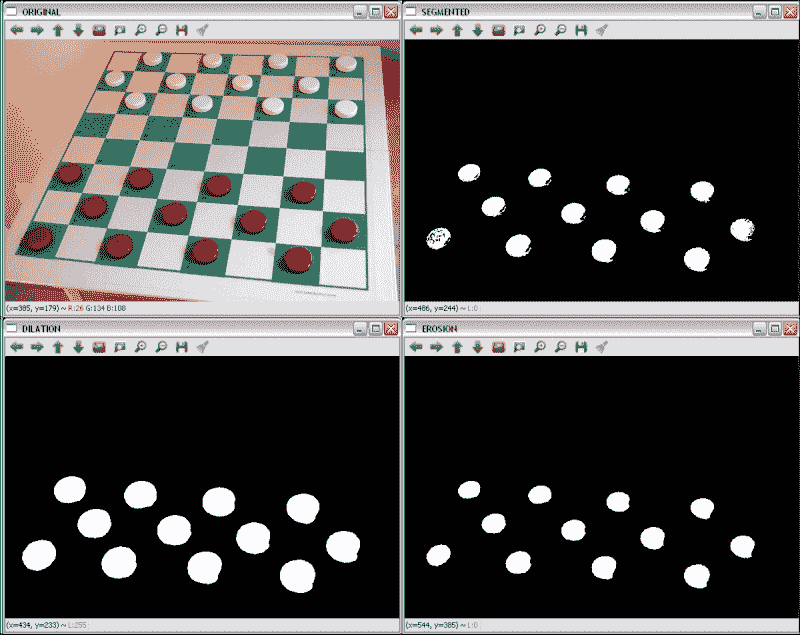# LUT

LUT(InputArray src, InputArray lut, OutputArray dst, int interpolation = 0) OpenCV 函数对 8 位有符号或src无符号图像应用查找表转换。 因此，lut参数中给出的表包含 256 个元素。 lut中的通道数为 1 或src.channels。 如果src具有多个通道，但lut具有单个通道，则将同一lut通道应用于所有图像通道。

## 示例代码

    uchar * M = (uchar*)malloc(256*sizeof(uchar));
for(int i=0; i<256; i++){
M[i] = i*0.5; //The result is rounded to an integer value
}
Mat lut(1, 256, CV_8UC1, M);


#include "opencv2/opencv.hpp"

using namespace cv;

int main( int argc, char** argv )
{
Mat src;

// Create the LUT
uchar * M = (uchar*)malloc(256*sizeof(uchar));
for(int i=0; i<256; i++){
M[i] = i*0.5;
}
Mat lut(1, 256, CV_8UC1, M);

// Apply the LUT
Mat dst;
LUT(src,lut,dst);

// Show the results
namedWindow( " ORIGINAL ", WINDOW_AUTOSIZE );
imshow( " ORIGINAL ", src );
namedWindow( " LUT ", WINDOW_AUTOSIZE );
imshow( " LUT ", dst );

waitKey();
return 0;
}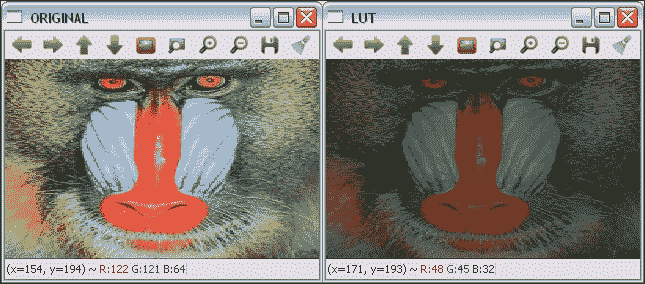# 几何变换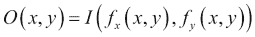• 外推f[x](x, y)f[y](x, y)可获得表示图像外部边界像素的值。 几何变换中使用的外推方法与图像过滤中使用的外推方法相同，外加另一种称为BORDER_TRANSPARENT的方法。
• 插值f[x](x, y)f[y](x, y)通常是浮点数。 在 OpenCV 中，可以在最近邻和多项式插值方法之间进行选择。 最近邻插值包括将浮点坐标舍入到最接近的整数。 支持的插值方法是：
• INTER_NEAREST：这是前面解释的最近邻插值。
• INTER_LINEAR：这是一种双线性插值方法。 默认情况下使用。
• INTER_AREA：使用像素面积关系重新采样。
• INTER_CUBIC：这是在4 x 4像素邻域上的双三次插值方法。
• INTER_LANCZOS4：这是在8 x 8像素邻域上的 Lanczos 插值方法。

OpenCV 支持的几何变换包括仿射（缩放，平移，旋转等）和透视变换。

## 仿射变换

### 缩放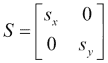#### 示例代码

#include "opencv2/opencv.hpp"

using namespace cv;

int main( int argc, char** argv )
{
Mat src;

// Apply the scale
Mat dst;
resize(src, dst, Size(0,0), 0.5, 0.5);

// Show the results
namedWindow( " ORIGINAL ", WINDOW_AUTOSIZE );
imshow( " ORIGINAL ", src );
namedWindow( " SCALED ", WINDOW_AUTOSIZE );
imshow( " SCALED ", dst );

waitKey();
return 0;
}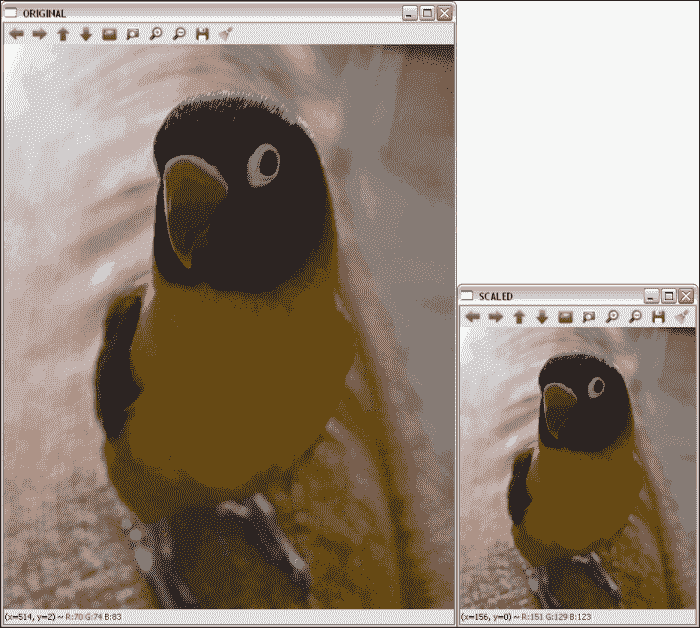### 平移#### 示例代码

#include "opencv2/opencv.hpp"

using namespace cv;

int main( int argc, char** argv )
{
Mat src;

// Apply translation
Mat dst;
Mat M = (Mat_<double>(2,3) << 1, 0, 200, 0, 1, 150);
warpAffine(src,dst,M,src.size());

// Show the results
namedWindow( " ORIGINAL ", WINDOW_AUTOSIZE );
imshow( " ORIGINAL ", src );
namedWindow( " TRANSLATED ", WINDOW_AUTOSIZE );
imshow( " TRANSLATED ", dst );

waitKey();
return 0;
}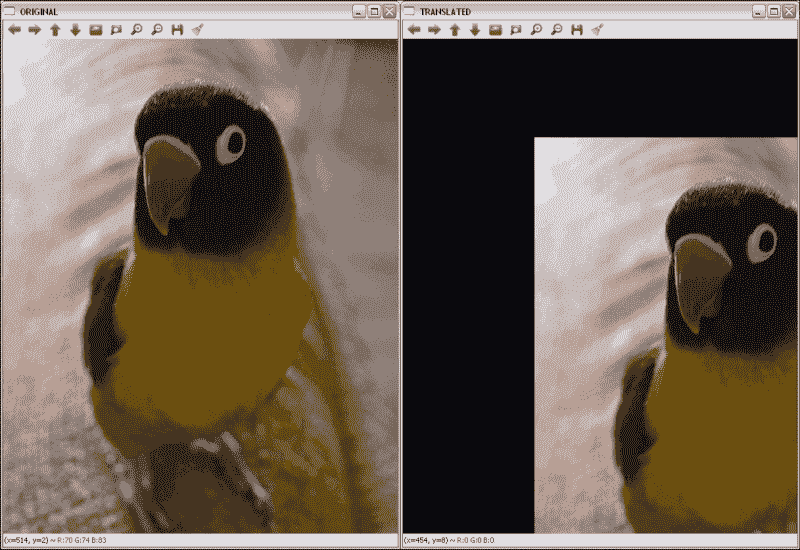### 图像旋转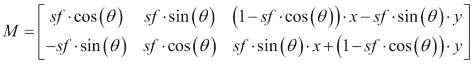#### 示例代码

#include "opencv2/opencv.hpp"

using namespace cv;

int main( int argc, char** argv )
{
Mat src;

// Apply the rotation
Mat dst;
Mat M = getRotationMatrix2D(Point2f(src.cols/2,src.rows/2),45,1);
warpAffine(src,dst,M,src.size());

// Show the results
namedWindow( " ORIGINAL ", WINDOW_AUTOSIZE );
imshow( " ORIGINAL ", src );
namedWindow( " ROTATED ", WINDOW_AUTOSIZE );
imshow( " ROTATED ", dst );

waitKey();
return 0;
}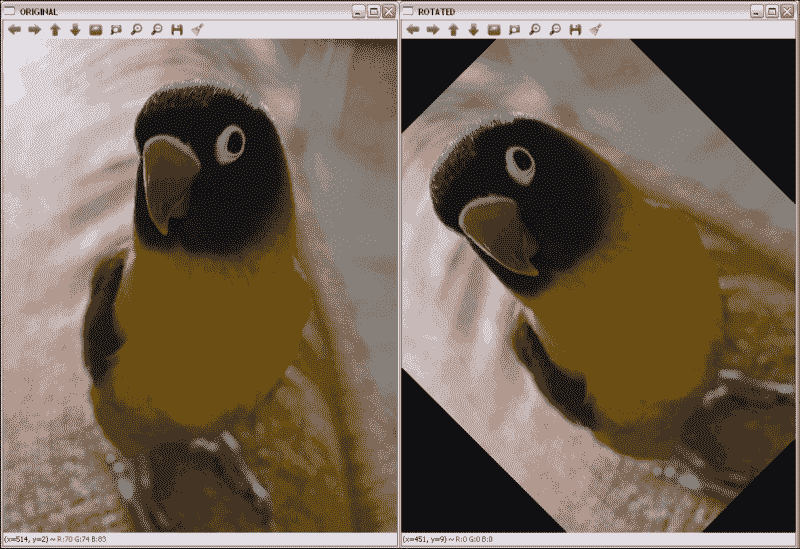### 倾斜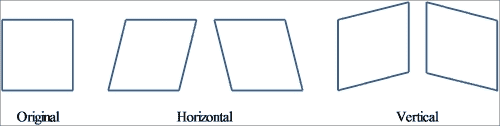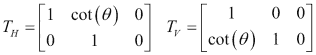### 提示

#### 示例代码

#include "opencv2/opencv.hpp"
#include <math.h>

using namespace cv;

int main( int argc, char** argv )
{
Mat src;

// Apply skew
Mat dst;
double m = 1/tan(M_PI/3);
Mat M = (Mat_<double>(2,3) << 1, m, 0, 0, 1, 0);
warpAffine(src,dst,M,Size(src.cols+0.5*src.cols,src.rows));

// Show the results
namedWindow( " ORIGINAL ", WINDOW_AUTOSIZE );
imshow( " ORIGINAL ", src );
namedWindow( " SKEWED ", WINDOW_AUTOSIZE );
imshow( " SKEWED ", dst );

waitKey();
return 0;
}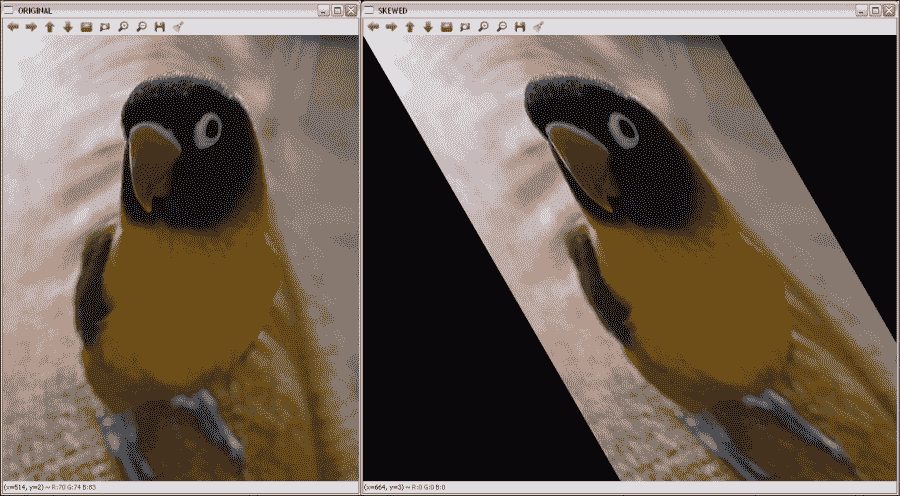### 反射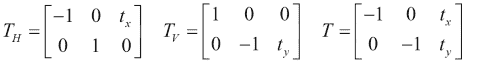### 注意

#### 示例代码

#include "opencv2/opencv.hpp"

using namespace cv;

int main( int argc, char** argv )
{
Mat src;

// Apply the reflections
Mat dsth, dstv, dst;
Mat Mh  = (Mat_<double>(2,3) << -1, 0, src.cols, 0, 1, 0
Mat Mv  = (Mat_<double>(2,3) << 1, 0, 0, 0, -1, src.rows);
Mat M  = (Mat_<double>(2,3) << -1, 0, src.cols, 0, -1, src.rows);
warpAffine(src,dsth,Mh,src.size());
warpAffine(src,dstv,Mv,src.size());
warpAffine(src,dst,M,src.size());

// Show the results
namedWindow( " ORIGINAL ", WINDOW_AUTOSIZE );
imshow( " ORIGINAL ", src );
namedWindow( " H-REFLECTION ", WINDOW_AUTOSIZE );
imshow( " H-REFLECTION ", dsth );
namedWindow( " V-REFLECTION ", WINDOW_AUTOSIZE );
imshow( " V-REFLECTION ", dstv );
namedWindow( " REFLECTION ", WINDOW_AUTOSIZE );
imshow( " REFLECTION ", dst );

waitKey();
return 0;
}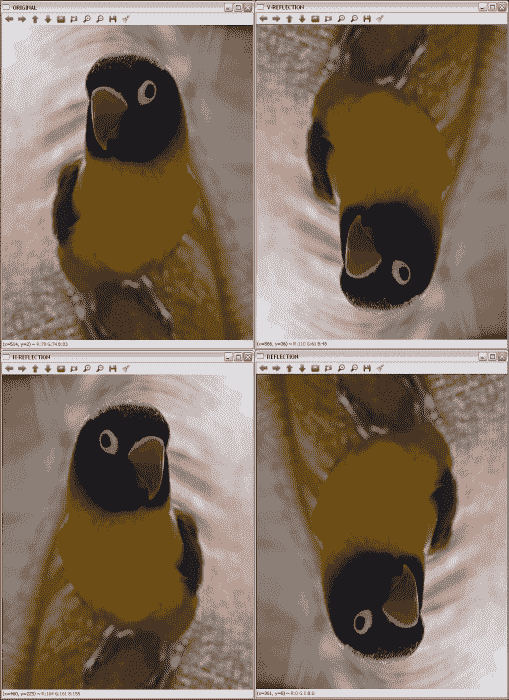X，Y 和两个轴上的原始图像和旋转图像

## 透视变换

• Mat getPerspectiveTransform(InputArray src, InputArray dst)Mat getPerspectiveTransform(const Point2f src[], const Point2f dst[])：这将返回根据srcdst计算的透视变换矩阵。
• void warpPerspective(InputArray src, OutputArray dst, InputArray M, Size dsize, int flags=INTER_LINEAR, int borderMode=BORDER_CONSTANT, const Scalar& borderValue=Scalar())：这会将M仿射变换应用于src图像，从而获得新的dst图像。 其余参数与讨论的其他几何变换相同。

### 示例代码

    Point2f src_verts;
src_verts = Point(195, 140);
src_verts = Point(410, 120);
src_verts = Point(220, 750);
src_verts = Point(400, 750);
Point2f dst_verts;
dst_verts = Point(160, 100);
dst_verts = Point(530, 120);
dst_verts = Point(220, 750);
dst_verts = Point(400, 750);


#include "opencv2/opencv.hpp"

using namespace cv;

int main( int argc, char** argv )
{
Mat src;

Mat dst;
Point2f src_verts;
src_verts = Point(195, 140);
src_verts = Point(410, 120);
src_verts = Point(220, 750);
src_verts = Point(400, 750);
Point2f dst_verts;
dst_verts = Point(160, 100);
dst_verts = Point(530, 120);
dst_verts = Point(220, 750);
dst_verts = Point(400, 750);

// Obtain and Apply the perspective transformation
Mat M = getPerspectiveTransform(src_verts,dst_verts);
warpPerspective(src,dst,M,src.size());

// Show the results
namedWindow( " ORIGINAL ", WINDOW_AUTOSIZE );
imshow( " ORIGINAL ", src );
namedWindow( " PERSPECTIVE ", WINDOW_AUTOSIZE );
imshow( " PERSPECTIVE ", dst );

waitKey();
return 0;
}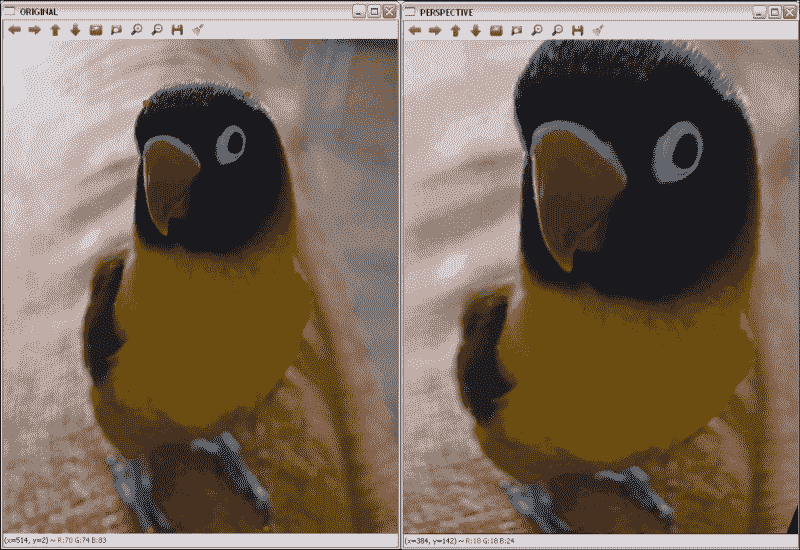# 修复

OpenCV 从版本 2.4 开始支持修复算法。 用于此目的的函数是：

• void inpaint(InputArray src, InputArray inpaintMask, OutputArray dst, double inpaintRadius, int flags)：这将恢复源（src）图像中inpaintMask参数用非零值指示的区域。 inpaintRadius参数指示flags指定的算法要使用的邻域。 OpenCV 中可以使用两种方法：
• INPAINT_NS：这是基于 Navier-Stokes 的方法
• INPAINT_TELEA：这是 Alexandru Telea 提出的方法

## 示例代码

#include "opencv2/opencv.hpp"

using namespace cv;

int main( int argc, char** argv )
{
Mat src;

// Apply the inpainting algorithms
Mat dst, dst2;

// Show the results
namedWindow( " ORIGINAL ", WINDOW_AUTOSIZE );
imshow( " ORIGINAL ", src );
namedWindow( " MASK ", WINDOW_AUTOSIZE );
namedWindow(" INPAINT_TELEA ", WINDOW_AUTOSIZE );
imshow( " INPAINT_TELEA ", dst );
namedWindow(" INPAINT_NS ", WINDOW_AUTOSIZE );
imshow( " INPAINT_NS ", dst2 );

waitKey();
return 0;
}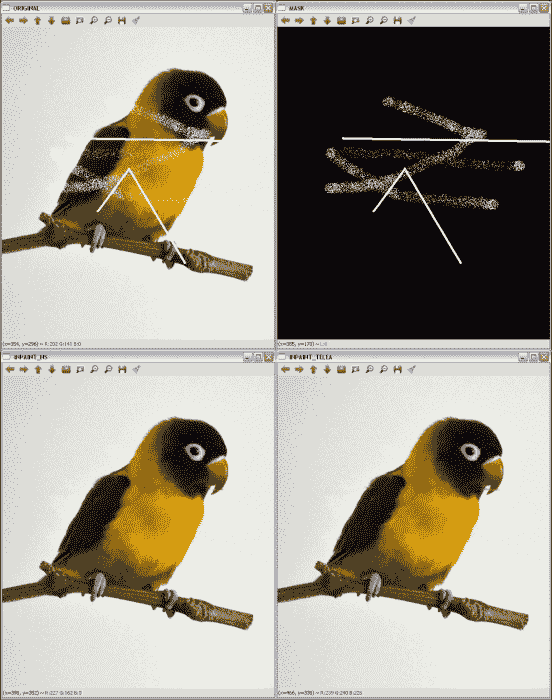### 注意

#include "opencv2/opencv.hpp"

using namespace cv;

int main( int argc, char** argv )
{
Mat src;

// Apply the inpainting algorithms
Mat dst, dst2;

// Show the results
namedWindow( " ORIGINAL ", WINDOW_AUTOSIZE );
imshow( " ORIGINAL ", src );
namedWindow( " MASK ", WINDOW_AUTOSIZE );
namedWindow(" INPAINT_TELEA ", WINDOW_AUTOSIZE );
imshow( " INPAINT_TELEA ", dst );
namedWindow(" INPAINT_NS ", WINDOW_AUTOSIZE );
imshow( " INPAINT_NS ", dst2 );

waitKey();
return 0;
}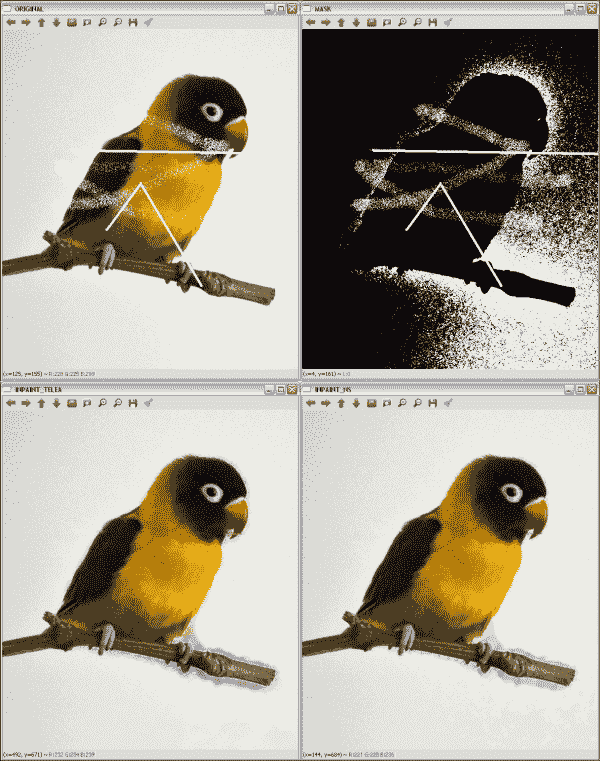# 降噪

### 注意

OpenCV 提供了四种使用非局部均值方法对彩色和灰度图像进行降噪的函数。 对于 TVL1 模型，提供了一种函数。 这些函数为：

• void fastNlMeansDenoising(InputArray src, OutputArray dst, float h = 3, int templateWindowSize = 7, int searchWindowSize = 21)：这会将src中加载的单个灰度图像降噪。 templateWindowSizesearchWindowSize 参数是用于计算权重的模板补丁的像素大小，以及用于计算给定像素的加权平均值的窗口大小。 这些应该是奇数，建议值分别为 7 和 21 像素。 h参数调节算法的效果。 较大的h值可消除更多的噪点缺陷，但具有消除更多图像细节的缺点。 输出存储在dst中。
• void fastNlMeansDenoisingColored(InputArray src, OutputArray dst, float h = 3, float hForColorComponents = 3, int templateWindowSize = 7, int searchWindowSize = 21)：这是对彩色图像先前函数的修改。 它将src图像转换为 CIELAB 色彩空间，然后使用fastNlMeansDenoising函数分别对 L 和 AB 分量进行降噪。
• void fastNlMeansDenoisingMulti(InputArrayOfArrays srcImgs, OutputArray dst, int imgToDenoiseIndex, int temporalWindowSize, float h = 3, int templateWindowSize = 7, int searchWindowSize = 21)：这使用图像序列获得去噪的图像。 在这种情况下，还需要两个参数：imgToDenoiseIndextemporalWindowSizeimgToDenoiseIndex的值是srcImgs中要去噪的目标图像索引。 最后，temporalWindowSize用于确定要用于降噪的周围图像的数量。 这应该很奇怪。
• void fastNlMeansDenoisingColoredMulti(InputArrayOfArrays srcImgs, OutputArray dst, int imgToDenoiseIndex, int temporalWindowSize, float h = 3, float hForColorComponents = 3, int templateWindowSize = 7, int searchWindowSize = 21)：基于fastNlMeansDenoisingColoredfastNlMeansDenoisingMulti函数。 这些参数将在其余函数中说明。
• void denoise_TVL1(const std::vector<Mat>& observations, Mat& result, double lambda, int niters)：这从observations中存储的一个或多个噪声图像获得result中的去噪图像。 lambdaniters参数控制算法的强度和迭代次数。

## 示例代码

Mat noisy = src.clone();
Mat noise(src.size(), src.type());
randn(noise, 0, 50);
noisy += noise;


#include "opencv2/opencv.hpp"

using namespace cv;

int main( int argc, char** argv )
{
Mat src;

Mat noisy = src.clone();
Mat noise(src.size(), src.type());
randn(noise, 0, 50);
noisy += noise;

// Apply the denoising algorithm
Mat dst;
fastNlMeansDenoisingColored(noisy, dst,30,30,7,21);

// Show the results
namedWindow( " ORIGINAL ", WINDOW_AUTOSIZE );
imshow( " ORIGINAL ", src );
namedWindow( " ORIGINAL WITH NOISE ", WINDOW_AUTOSIZE );
imshow( " ORIGINAL WITH NOISE ", noisy );
namedWindow(" DENOISED ", WINDOW_AUTOSIZE );
imshow( " DENOISED ", dst );

waitKey();
return 0;
}